# Exterior Orientation

## Producer Field Guide

HGD_Product
Producer Field Guide
HGD_Portfolio_Suite
Producer

Exterior orientation defines the position and angular orientation associated with an image. The variables defining the position and orientation of an image are referred to as the elements of exterior orientation. The elements of exterior orientation define the characteristics associated with an image at the time of exposure or capture.

The positional elements of exterior orientation include:

• Xo
• Yo
• Zo

They define the position of the perspective center (O) with respect to the ground space coordinate system (X, Y, and Z). Zo is commonly referred to as the height of the camera above sea level, which is commonly defined by a datum.

The angular or rotational elements of exterior orientation describe the relationship between the ground space coordinate system (X, Y, and Z) and the image space coordinate system (x, y, and z).

Three rotation angles are commonly used to define angular orientation:

• omega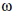-- a rotation about the photographic x-axis
• phi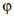-- a rotation about the photographic y-axis
• kappa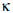-- a rotation about the photographic z-axis

Omega, phi, and kappa are defined as being positive if they are counterclockwise when viewed from the positive end of their respective axis.

The figure below illustrates the elements of exterior orientation.

Elements of Exterior Orientation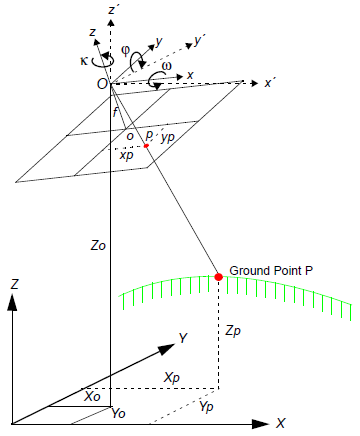Different conventions are used to define the order and direction of the three rotation angles (Wang, Z., 1990). The ISPRS recommends the use of the convention,.,.

The photographic z-axis is equivalent to the optical axis (focal length). The x', y', and z' coordinates are parallel to the ground space coordinate system.

Using the three rotation angles, the relationship between the image space coordinate system (x, y, and z) and ground space coordinate system (X, Y, and Z or x', y', and z') can be determined. A 3 x 3 matrix defining the relationship between the two systems is used. This is referred to as the orientation or rotation matrix, M. The rotation matrix can be defined as follows: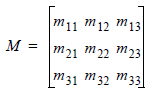The rotation matrix is derived by applying a sequential rotation of omega about the x-axis, phi about the y-axis, and kappa about the z-axis.

Collinearity Equation

The following section defines the relationship between the camera or sensor, the image, and the ground. Most photogrammetric tools utilize the following formulations in one form or another.

With reference to the "Elements of Exterior Orientation" figure above, an image vector a can be defined as the vector from the exposure station O to the image point p. A ground space or object space vector A can be defined as the vector from the exposure station O to the ground point P. The image vector and ground vector are collinear, inferring that a line extending from the exposure station to the image point and to the ground is linear.

The image vector and ground vector are only collinear if one is a scalar multiple of the other. Therefore, the following statement can be made: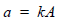Where k is a scalar multiple. The image and ground vectors must be within the same coordinate system. Therefore, image vector a is comprised of the following components: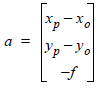Where xo and yo represent the image coordinates of the principal point.

Similarly, the ground vector can be formulated as follows: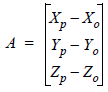In order for the image and ground vectors to be within the same coordinate system, the ground vector must be multiplied by the rotation matrix M. The following equation can be formulated: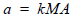Where: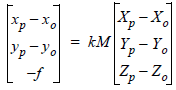The above equation defines the relationship between the perspective center of the camera or sensor exposure station and ground point P appearing on an image with an image point location of p. This equation forms the basis of the collinearity condition that is used in most photogrammetric operations. The collinearity condition specifies that the exposure station, ground point, and its corresponding image point location must all lie along a straight line, thereby being collinear. Two equations comprise the collinearity condition.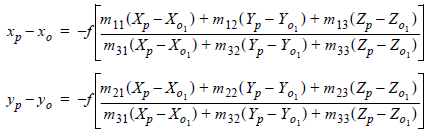One set of equations can be formulated for each ground point appearing on an image. The collinearity condition is commonly used to define the relationship between the camera or sensor, the image, and the ground.